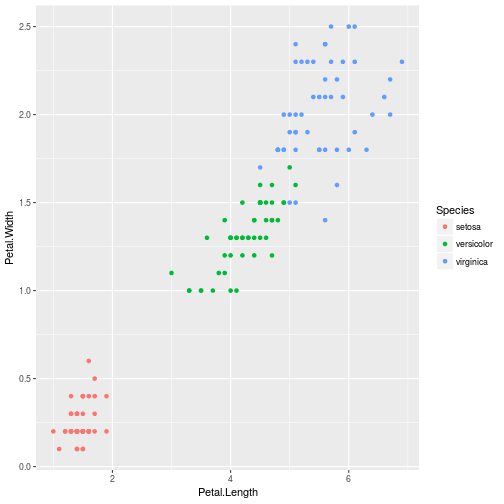# Introduction to Machine Learning in R

Slides are available at this link

## Demo examples:

### Linear regression

``````#install.packages("lattice")
library(lattice)
alligator <- data.frame(
lnLength = c(3.87, 3.61, 4.33, 3.43, 3.81, 3.83, 3.46, 3.76,
3.50, 3.58, 4.19, 3.78, 3.71, 3.73, 3.78),
lnWeight = c(4.87, 3.93, 6.46, 3.33, 4.38, 4.70, 3.50, 4.50,
3.58, 3.64, 5.90, 4.43, 4.38, 4.42, 4.25)
)

# Plot our information
plot(
alligator\$lnWeight,
alligator\$lnLength,
col = "blue",
xlab = "Snout vent length (inches) on log scale",
ylab = "Weight (pounds) on log scale",
main = "Alligators in Central Florida"
)
``````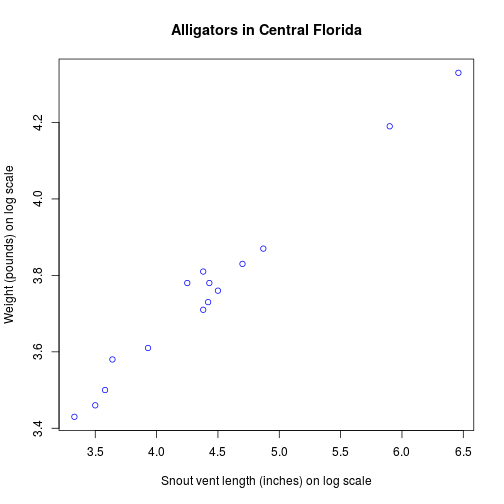lm function fits a linear model. A typical model has the form response ~ terms

``````myModel <- lm(lnLength ~ lnWeight, data = alligator)
``````

Visualize the regression

``````# Plot the chart with lm.
plot(
alligator\$lnWeight,
alligator\$lnLength,
col = "blue",
abline(myModel, col = 'red'),
xlab = "Snout vent length (inches) on log scale",
ylab = "Weight (pounds) on log scale",
main = "Alligators in Central Florida"
)
``````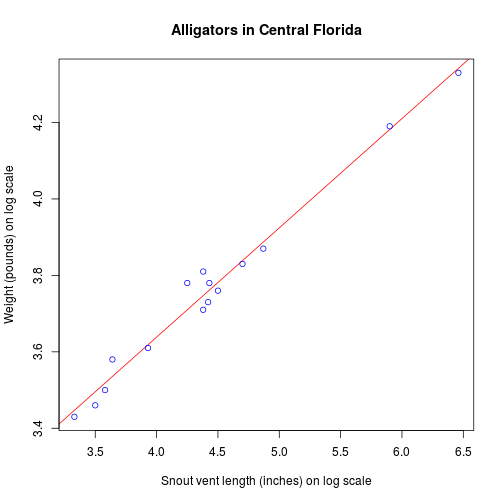``````# Find weight of a alligator with weight of 5.5.
find_value <- data.frame(lnWeight = 5.5)
result <- predict(myModel, find_value)
result
``````
``````##        1
## 4.067312
``````

If we trace the line with the value we are looking for we can see that the value seems correct

``````library(ggplot2)
end <- ggplot(alligator, aes(x = lnWeight, y = lnLength)) +
geom_point()
end + geom_smooth(method = 'lm', se = FALSE) +
geom_vline(xintercept = 5.5) +
geom_hline(yintercept = 4.06312, color = 'red')
``````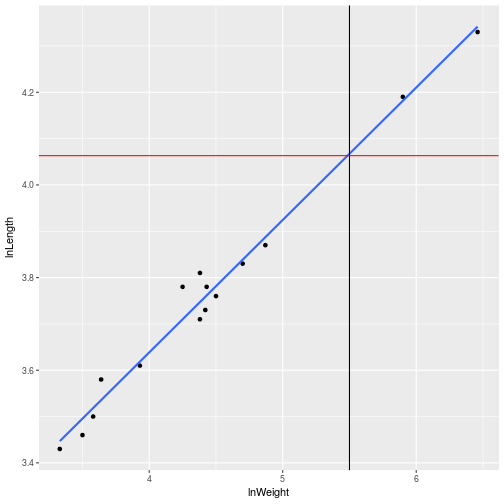### k-means

``````library(datasets)
head(iris)
``````
``````##   Sepal.Length Sepal.Width Petal.Length Petal.Width Species
## 1          5.1         3.5          1.4         0.2  setosa
## 2          4.9         3.0          1.4         0.2  setosa
## 3          4.7         3.2          1.3         0.2  setosa
## 4          4.6         3.1          1.5         0.2  setosa
## 5          5.0         3.6          1.4         0.2  setosa
## 6          5.4         3.9          1.7         0.4  setosa
``````
``````ggplot(iris, aes(Petal.Width, Petal.Length)) +
geom_point()
``````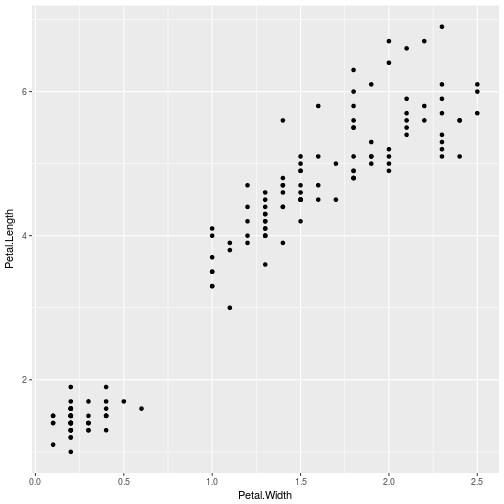After resetting the seed I do a kmeans just selecting the 3-4 columns of iris, collect 3 iterations, selecting 20 random sets

``````set.seed(20)
irisCluster <- kmeans(iris[, 3:4], 3, nstart = 20)
#Comparing cluster with species
table(irisCluster\$cluster, iris\$Species)
``````
``````##
##     setosa versicolor virginica
##   1     50          0         0
##   2      0         48         4
##   3      0          2        46
``````

We can graph our result

``````irisCluster\$cluster <- as.factor(irisCluster\$cluster)
ggplot(iris, aes(Petal.Length, Petal.Width, color = irisCluster\$cluster)) +
geom_point()
``````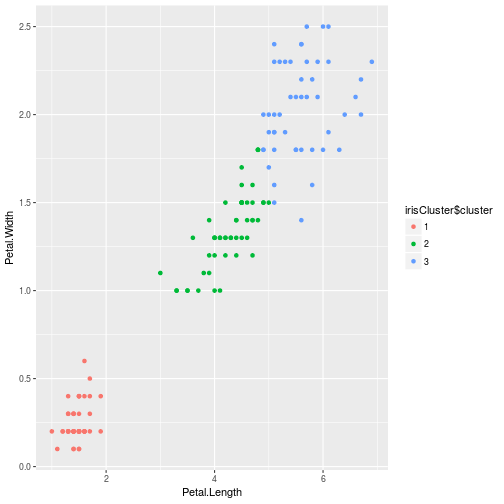Why did we do 3 clusters and not 4? dataset only has 3 species

``````ggplot(iris, aes(Petal.Length, Petal.Width, color = Species)) +
geom_point()
``````